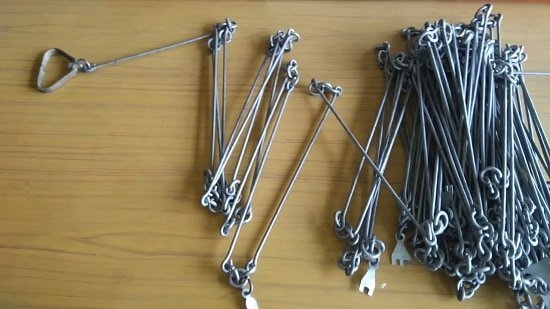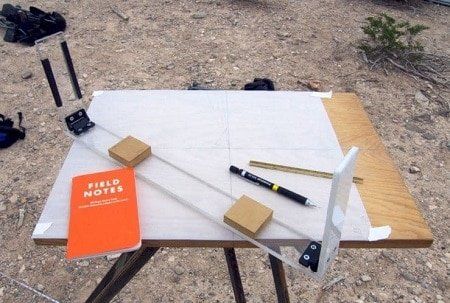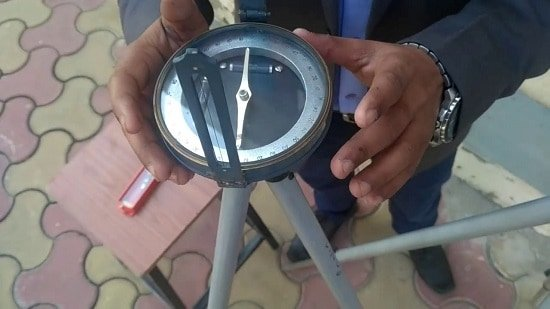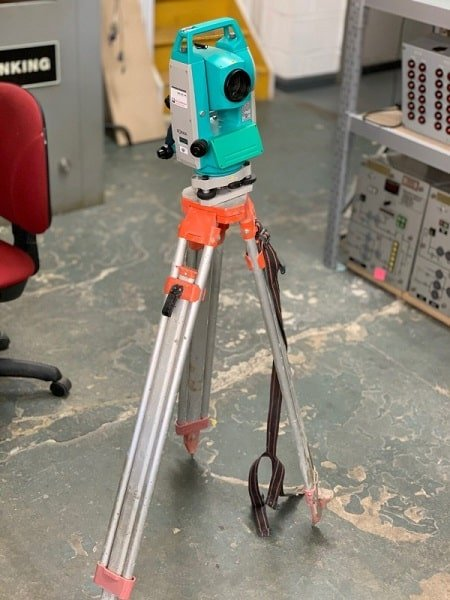# Types Of Surveying – Classification Of Surveying

Contents

## What Is Surveying?

Surveying is a scientific method that determines the three-dimensional positions and angles of relative points on the surface of the earth. Different types of surveying methods and their classification are used in construction which is described below.

## Types Of Surveying:

Surveying may be divided into two general categories.

1. Geodetic Surveying and
2. Plane Surveying.

### 1. Geodetic Surveying:

Geodetic surveying is a particular type of surveying where the curvature of the earth is taken into account. Since the earth has a spherical shape, the line connecting any two points on the earth’s surface is curved or is an arc.

Hence it involves spherical trigonometry. In geodetic surveying, large distances and areas are measured and its degree of accuracy is comparatively high.

### 2. Plane Surveying:

Plane surveying is a specific type of surveying where the surface of the earth is considered as a plane and the curvature of the earth is not taken into account. The line connecting any two points is a straight line and the angles of polygons are plane angles.

This type of surveying is suitable for small and flat areas, and its degree of accuracy is comparatively low. In America, the limit for treating a surface as a plane is about 250 km2.

Note: The difference in length between the arc and the subtended chord on the surface of the earth is only about 0.1 m in 18.2 km, 0.3 m in 54.3 km, and 0.5 m in 91 km respectively.

## Classification Of Surveying:

### A. Classification based on the nature of the field:

1. Land Surveying.
2. Marine Surveying.
3. Astronomical Surveying.

Land surveying can be sub-divided into the following categories:

• Topographical Surveys.
• City Surveys.
• Engineering Surveys.

Land surveying mainly deals with the natural and artificial features of a country such as hills, rivers, buildings, towns, villages, etc.

### B. Classification based on the object of the survey:

1. Archaeological survey.
2. Geological Survey.
3. Mine Survey.
4. Military Survey.

### C. Classification based on the instruments :

#### 1. Chain Surveying:

Chain surveying is the simplest method of surveying in which the linear measurements are directly taken in the field and the angular measurements are not taken. This type of surveying is used over small and leveled areas.#### 2. Plane Table Surveying:

In plane table surveying, the fieldwork and plotting are done using a graphical method. It is mostly adapted for small and medium scale mapping where great accuracy is not required.#### 3. Compass Surveying:

In this type of surveying, a compass is used to determine the direction of survey lines, and the length of survey lines are measured by a chain or tape, or laser range finder. The compass is generally used to run a traverse line. There are three types of compass usedn in surveying:

• Prismatic compass
• Surveyor’s compass
• Level compass#### 4. Tacheometric Surveying:

Tacheometric surveying is a method of surveying in which the horizontal and vertical distances of relative points are determined without using any sophisticated instruments such as chain, tape, etc.

#### 5. Theodolite Surveying:

The theodolite is an instrument that is used to measure the horizontal and vertical angles. It allows surveyors to “triangulate” the position of objects in a specific area. Theodolite are two types i.e transit theodolite and non-transit theodolite. Theodolite surveying are commonly used for

i) Prolongation of survey lines,

ii) Finding the difference in elevation, and

iii) Setting out engineering works that requires higher precision, i.e. ranging highway and railway curves, aligning tunnels, etc.#### 6. Photographic and Aerial Surveying:

These types of surveying are done by taking photographs from elevated ground stations. It deals with the production of maps such as topographic or planimetric maps by compiling number of photographs taken in that area.

### D. Classification based on the methods of Survey:

1. Triangulation Surveying

Triangulation is a method of surveying that measures the angles in a triangle formed by three survey control points. Using the trigonometry formula and the measured one side’s length, the other distances in the triangle are calculated respectively.

2. Traverse Surveying

It is also a well known method of surveying. A traverse is a series of connected lines whose lengths and direction are measured with the help of an angle measuring instrument and tape or chain respectively.

##### Types Of Curves In Surveying

Types Of Scales Used In Surveying

Plane Surveying Vs Geodetic Surveying

### 5 thoughts on “Types Of Surveying – Classification Of Surveying”

1. I REAL EXITED IN SURVEY IN GENERAL.